# AP Statistics : Mean and Linear Regression

## Example Questions

### Example Question #1 : How To Test Of Significance For The Slope Of A Least Squares Regression Line

A statistician conducts a regression analysis and obtains a p-value of 0.1. It is more likely than not that there is a relationship between the variables in the study.

False

True

True

Explanation:

A p-value of 0.1 is generally not sufficient to reject the null hypothesis, but this is only because we want a high degree of confidence before finding a relationship between variables.  Here, there is most likely a relationship between the variables even though the statistician could not reject the null hypothesis.

### Example Question #1 : How To Test Of Significance For The Slope Of A Least Squares Regression Line

For a data set, the least-squares regression line has a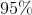confidence interval for the slope of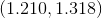.

Based on this confidence interval, what can you do with a hypothesis test at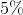significance level where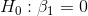and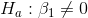?

Reject the null hypothesis because this confidence interval does not include.

Reject the null hypothesis because this confidence interval does not include.

Fail to reject the null hypothesis because this confidence interval does not include.

Fail to reject the null hypothesis because this confidence interval does not include.

Not enough information to be able to decide.

Reject the null hypothesis because this confidence interval does not include.

Explanation:

Notice that the interval does not include. This means that the P-value for the hypothesis test would be under 5%, which would lead us to reject our null hypothesis.

Any confidence interval can be used to create a hypothesis test by inverting it, and it is fairly simple, but the concept is tested into graduate-level statistics theory.

### Example Question #1 : Significance

Which of the following is an incorrect condition requirement for regression inference?

A linear relationship betweenandThe standard deviation of the response must be constan

Ordered pairs must be independent of each other

Response must vary normally about the regression line for any given value ofA trend/pattern of some sort in the residual plot

A trend/pattern of some sort in the residual plot

Explanation:

All of the following choices are correct conditions except for the choice concerning a trend/pattern of some sort in the residual plot. For regression inference to be accurate, we need to look at the residual plot of the data of interest and make sure there is random scatter. Random scatter indicates that the ordered pairs are indeed independent of each other. Any sort of pattern present in the residual plot would not satisfy that requirement, and therefore would not enable us to successfully use regression inference.

Explanation:

### Example Question #1 : Inference

Suppose you conduct a paired-test to assess whether two group means significantly differ and find a-score of 1.645. At what alpha level would this cause you to reject the null?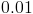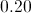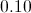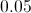Explanation:

The critical value for a-test with alpha set to 0.10 is 1.645.

### Example Question #1 : Inference

Bob wants to statistically determine if the mean height of middle school boys is greater than the mean height of middle school girls. He wants to use a significance level of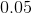What must be true for him to reject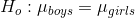.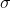is known.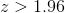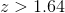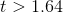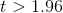Explanation:

Step 1: We need to use a 2-sample z test because there are 2 samples, boys and girls. The population standard deviation,, is known, so we can assume a standard distribution for each sample.

Step 2: This is a one-sided z test because the questions asks if the mean height of boys is greater than the mean height of girls.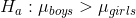Step 3: significance level, or alpha, is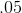. This means we need a p-value less thanin order to reject the null hypothesis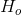. A z-score greater than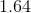would ensure a p-value less than.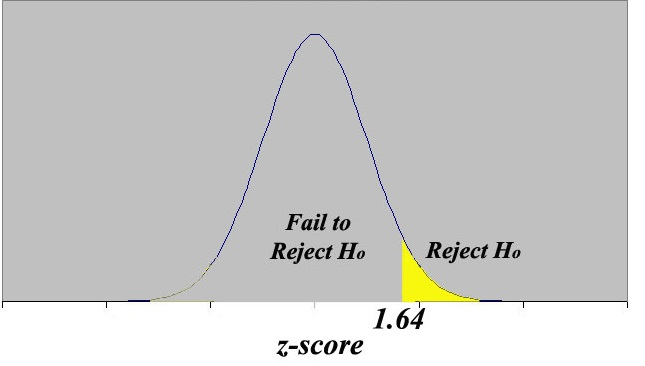### All AP Statistics Resources